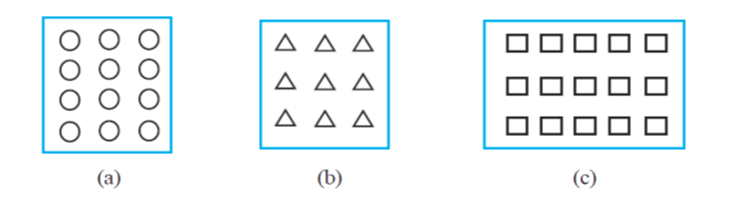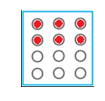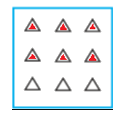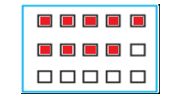# Ex 2.2 Q4 Fractions-and-Decimals-Solutions NCERT Maths Class 7

Go back to  'Ex.2.2'

## Question

(i) \begin{align}\frac{1}{2}\end{align} of the circles in box (a)

(ii) \begin{align}\frac{2}{3}\end{align} of the triangles in box (b)

(iii) \begin{align}\frac{3}{5}\end{align} of the squares in box (c)Video Solution
Fractions And Decimals
Ex 2.2 | Question 4

## Text Solution

What is known?

What is unknown?

Shaded figure according to given fraction.

Reasoning:

First multiple the fraction by number of parts of given figure that’s how we will get what number of parts need to be shade.

Steps:

(i) Total number of circles in box $$(a) = 12$$

\begin{align}\frac{1}{2}\text{ of circles}&=\frac{1}{2} \times 12 \\&= 6\, \text{circles}\end{align}

So, $$6$$ circles should be shaded(ii) Total number of triangles in box $$(b) = 9$$

\begin{align}\frac{2}{3} \text{ of triangles} &=\frac{2}{3} \times 9 \\&= 6 \,\text{triangles}\end{align}

So, $$6$$ triangles should be shaded.iii) Total number of squares in box $$(c) = 15$$

\begin{align}\frac{3}{5} \,{\rm of }\,\times 15\, \text{squares} &= \frac{3}{5} \times 15 \\&= 3 \times 3 \\&= 9 \text{ squares}\end{align}

So, $$9$$ squares should be shadedLearn from the best math teachers and top your exams

• Live one on one classroom and doubt clearing
• Practice worksheets in and after class for conceptual clarity
• Personalized curriculum to keep up with school